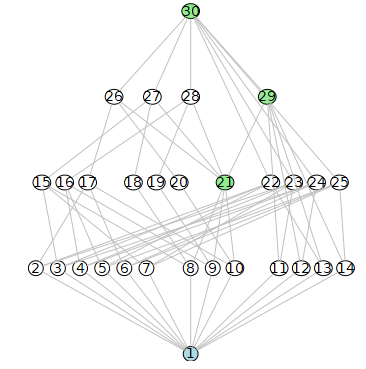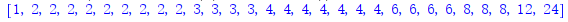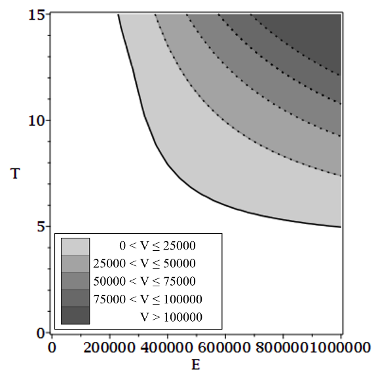## Difficulty with concatenation and indexing...

Why this error message ?
with(geometry);
vartheta := (2*Pi)/17;
x || k := cos(k*theta);
y || k := sin(k*theta);
xk := cos(k theta): yk := sin(k theta)
.M||k:=(point,x||k,y||k);
with(plots):
Points := pointplot([seq(M || k, k = 1 .. 16)], symbol = solidcircle, color = red, symbolsize = 10):
Error, (in plots:-pointplot) cannot convert data to an Array of datatype float; Thank you for your answer..

## How do I put this linear transformation in Maple? ...

Hello, I have a question.The exercise consists of defining the image in relation to a chosen base. For example, I choose {x^4,x^3*y,x^2*y^2,x*y^3,y^4} as a base. Then I want to calculate T(x^4), T(x^3*y),T(x^2*y^2),T(x*y^3),T(y^4) through maple but but maple won't calculate it.Do you know what I'm doing wrong?

## How to solve a cubic assuming discriminant conditi...

Hi MaplePrimes.

I have a cubic with some parameters that I would like to solve and recieve 3 real solutions, however I cannot seem to find a way to get that to work while using assumptions. I always recieve 1 real and two complex conjugate answers. I have tried assuming the discrimant to be greater then zero but nothing seems to be working, and I have looked through a few posts on here but I cant seem to find anything out. In particular solve({f,conditions},{variable})

If I solve with a known set of paramters(which i determine graphically) that gives three solutions everything works fine. Also if I solve the cubic generally and recieve the two complex solutions and one real then substitute the paramters in I get the same correct answers, so I am slightly baffled. If I have missed something simple my apologies.

Any help would be greatly appreciated. File attached.

CubicSolve.mw

## How to label the DrawSubgroupLattice with the orde...

This is the default style about the group of `DrawSubgroupLattice`:

`DrawSubgroupLattice(SymmetricGroup(4))`But I hope to label the `DrawSubgroupLattice` with the order of the subgroup but not the default index, is it possible? I can get the order with this code:

`GroupOrder~(SubgroupLattice(SymmetricGroup(4), output = list))`But I don't know how to label it into the node...

## Why plot(F(R), R = 0 .. 100) doesn't work?...

How to plot F(R) for R=0..100 ?

Maple 2021 sheet attached.(1)(2)(3)(4)plot(F(R), R = 0 .. 100)

## How to use the group produced with DirectProduct?...

I don't know how to use the group produced with `DirectProduct`. such as

```G := DirectProduct(QuaternionGroup(), CyclicGroup(3)):
DrawSubgroupLattice(G)```

Or

`IsNormal(CyclicGroup(2), DirectProduct(CyclicGroup(2), CyclicGroup(2)))`

They all will get error information. It looks like the format is different, do I need to convert it somehow? Or is this a bug in maple?

## coloring surfaces in a plot...

Hello,

Im a tax student and tring to figure out Maple. I plotted the following graph with the code:

implicitplot([Vth = Vps, V1 = 25000, V1 = 50000, V1 = 75000, V1 = 100000], E = 0 .. 1000000, T = 0 .. 15, color = [black], labels = ["E in (EUR)", "T (in Jahren)"], labelfont = [times, bold, 12], view = [0 .. 1000000, 0 .. 15])I would like to color the intermediate areas and attach a legend. A colleague has plotted this for me, but unfortunately has not provided me with the source code and has now lost the file. Can someone help me to re-plot the last shown chart?Thanks so so much in advance!! Best regards Rebekka